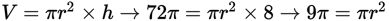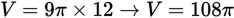# SAT Math Multiple Choice Question 985: Answer and Explanation

### Test Information

Question: 985

5. A cylinder has a volume of 72π cubic inches and a height of 8 inches. If the heightis increased by 4 inches, what will be the new volume of the cylinder in cubic inches?

• A. 76π
• B. 108π
• C. 328π
• D. 576π

Getting to the Answer: Plug the volume and height of the first cylinder into the correct volume formula to find the area of its base:. Notice that you don't need to solve for the radius, as it will remain constant. Plug the area of the base back into the volume formula with the new height, which is 8 + 4 = 12 inches:. (B) is correct.